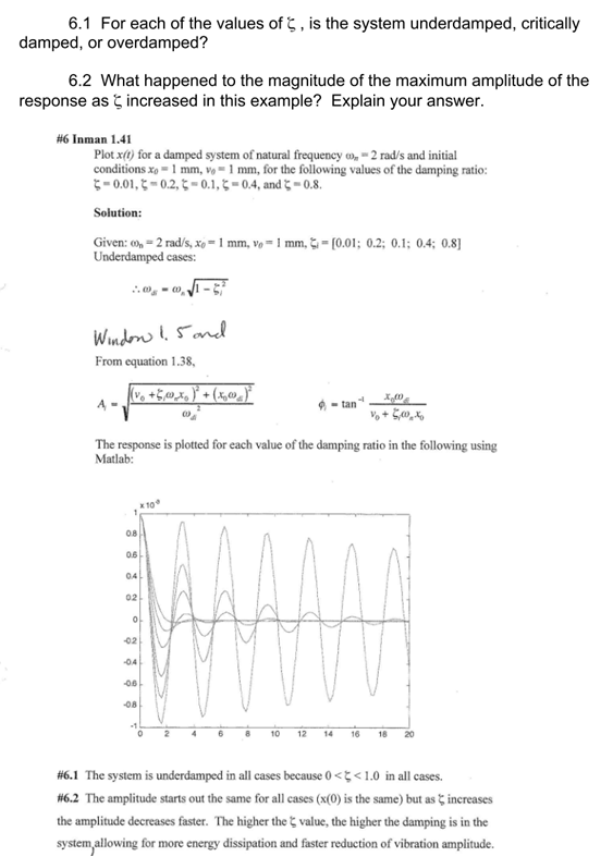Plot x(t) for a damped system of natural frequency wn=2 rad/s and initial conditions x0=1mm, v0=1mm, for the folloiwng values of the damping ratio: 0.01, 0.2, 0.1, 0.4, and 0.8. For each of the values of the damping ratio, is the system underdamped, critically damped, or overdamped? What happened to the magnitude of the maximum amplitude of the response as the damping ratio increased in this example? Explain your answer.Plot x(t) for a damped system of natural frequency wn=2 rad/s and initial conditions x0=1mm, v0=1mm, for the folloiwng values of the damping ratio: 0.01, 0.2, 0.1, 0.4, and 0.8. For each of the values of the damping ratio, is the system underdamped, critically damped, or overdamped? What happened to the magnitude of the maximum amplitude of the response as the damping ratio increased in this example? Explain your answer.

Vibrations Page 1 vibrations vibrations vibrations vibrations vibrations vibrations vibrations Vibrations Page 2